Home / Basic Electrical / AC Waveform & AC Circuit Theory

AC Waveform & AC Circuit Theory

Want create site? Find Free WordPress Themes and plugins.

Generally, the term Alternating Current (AC) is used to describe any current that periodically changes direction. For example, take a look at the circuit current time relationship as shown in Figure 1. The horizontal axis of the graph is used to represent time (t). The vertical axis of the graph is used to represent both Magnitude (value) and direction of the current. As the figure is shown, the current build to a peak value in one direction and returns to zero (t0 to t2). Note that the current not only changes direction but constantly changes to magnitude.Figure 1: Alternating Current (AC)

A graph of the relationship between magnitude and time is referred to as a waveform. The waveform in Figure 1 is called a Sinusoidal Waveform, or Sine Wave. It should be noted that the sine wave is not the only type of AC waveform.

Basic AC Operations

The operations of a simple AC circuit is illustrated in Figure 2. The Figure2a highlights the operation of the circuit during the positive half of the AC input. Figure 2b highlights the operation of the circuit during the negative half of the AC input. Using the time periods shown in the figure, the operation of the circuit can be described as follows:

 Time Period Circuit Operation t0 to t1 The output from the voltage source increases from 0V to its positive peak value (+V) peak. As the voltage increases, the current also increases from 0A to its peak value. Note the voltage polarity and the current direction are shown in the figure. t1 to t2 The output from the voltage source returns to 0V and the current returns to 0A. t2 to t3 The output voltage from the source increases to its negative peak value (-V) peak, as does the circuit current. Note that both the voltage polarity and the current direction have reversed. t3 to t4 The output from the voltage source returns to 0V and the current returns to 0A.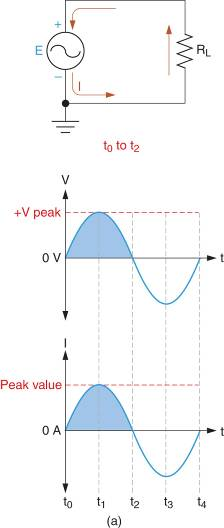Figure 2: AC Circuit Operation

Alternations and Cycles

The positive and negative halves of the AC waveform in Figure 2 are referred to as Alternations. The complete transition through one positive alternation and one negative alternation is referred to as a Cycle.

These terms are used to identify the parts of the AC waveform in Figure 3a. Since two alterations make up a cycle, each alternation is commonly referred to as a Half Cycle. As shown in Figure 3b, a sine wave is made of a continuous stream of cycles. A waveform that repeats itself continuously (like the one shown in Figure 3b) is called Periodic Waveform.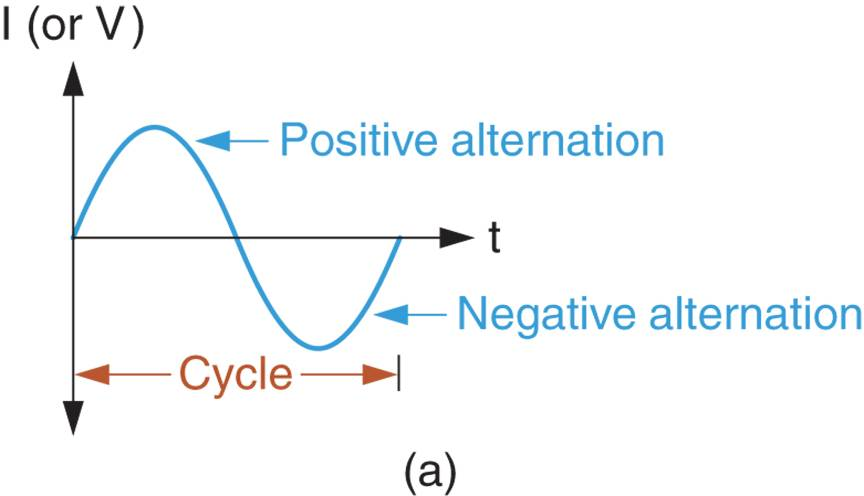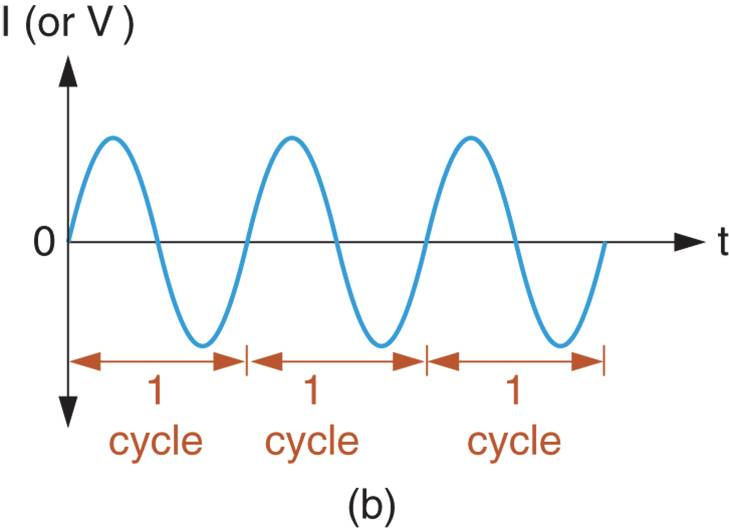Figure 3: Sine Wave Alterations and Cycles

Waveform Period and Frequency

The time required to complete one cycle of an AC waveform is referred to as its Period. Example 1 demonstrates the concept of waveform period.

Example 1

What is the period of the waveform represented in figure 3a?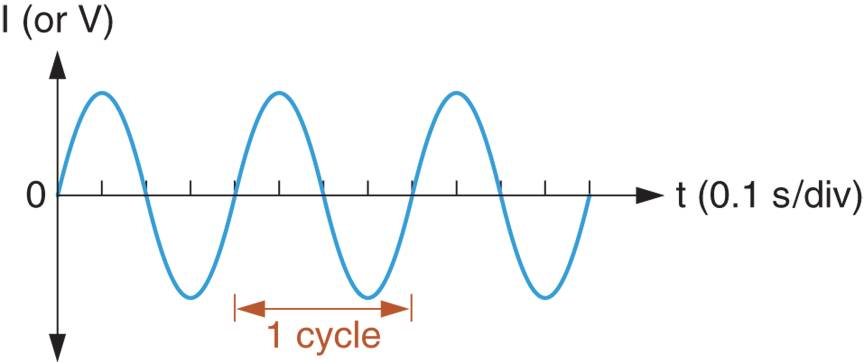Figure 3a

Solution

Each division on the horizontal scale of the graph represents a time period of 0.1s. One cycle of the waveform is four division in length. Therefore, the period (T) of the waveform is found as:

${{T}_{C}}=\left( 4\text{ }div \right)\times 0.1\frac{s}{div}=0.4s=400ms$

The period of the AC waveform can be measured from any given point on the waveform to the identical point in the next cycle, as illustrated in Figure 4. With a horizon scale of 20 ms/dv, the total cycle time (regardless of where it is measured) work out to be

${{T}_{C}}=\left( 4\text{ }div \right)\times 20\frac{ms}{div}=80ms$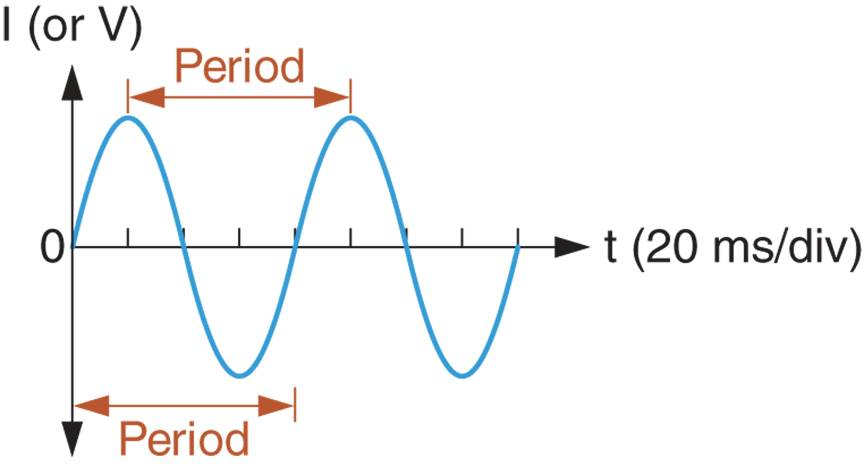Figure 4: AC Waveform Period Measurements

The frequency (f) of a waveform is the rate at which the cycles repeat themselves, in cycles per second. The concept of frequency is illustrated in Figure 5.Figure 5: Frequency and Time Period Calculation of a Waveform

The AC waveform shown has a cycle time of 200ms. Therefore, the cycle repeats itself five times every second, and the frequency (f) of the waveform is expressed as:

f = 5 cycles per second

The unit of measure for frequency is the Hertz (Hz). One hertz is equal to one cycle per second. Therefore, the frequency of the waveform in Figure 5 would be written as:

f = 5Hz.

When the cycle time of a waveform is known, the frequency of the waveform can be found as:

$\begin{matrix}f=\frac{1}{T} & {} & \left( 1 \right) \\\end{matrix}$

The use of this equation is demonstrated in Example 2.

Example 2

What is the frequency of a sine wave that has a period of 100 ms?

Solution

When the period of 100 ms, the sine wave has a frequency of

$f=\frac{1}{T}=\frac{1}{100\times {{10}^{-3}}s}=\text{10cycles per second}=10\text{ }Hz$

When the frequency of a waveform is known, its period can be found as:

$\begin{matrix}T=\frac{1}{f} & {} & \left( 2 \right) \\\end{matrix}$

The use of this relationship is demonstrated in Example 3.

Example 3

What is the period of a 400 Hz sine wave?

Solution

$T=\frac{1}{f}=\frac{1}{400Hz}=2.5ms$

Oscilloscope Time and Frequently Measurement

An Oscilloscope is a piece of test equipment that provides a visual representation of a voltage waveform. The visual display can be used to make a variety of magnitude and time-related measurements. An oscilloscope is shown in Figure 6, along with the display of the sine wave.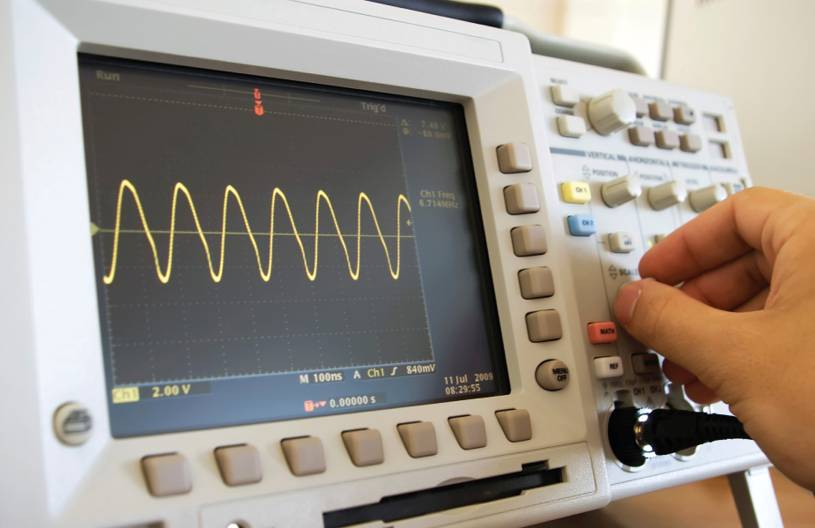Figure 6: An oscilloscope and sine wave display

The oscilloscope screen is divided into a series of major divisions and minor divisions, as shown in Figure 7. The divisions along the x-axis are used to measure time, while the divisions of y-axis are used to measure voltage.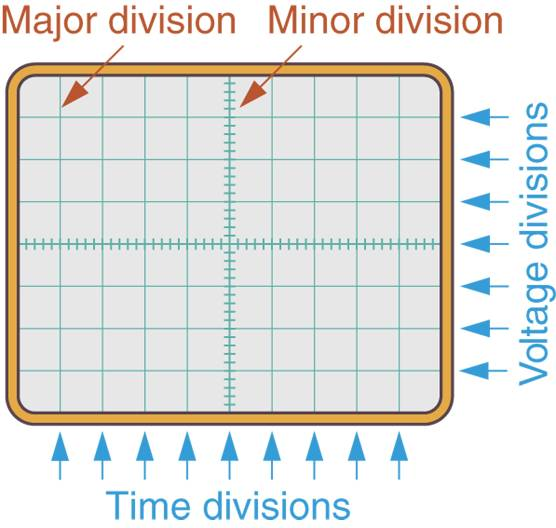Figure 7: An Oscilloscope Display Grid

The setting of the Time Base control on the oscilloscope determines the time represented by the interval between any two adjacent minor divisions along the x-axis. This point is illustrated in Figure 8a. If the time base control is set to 5ms/dv, the time intervals have the values shown. In each case, the time interval represented on the screen equals the time base setting times the number of major divisions.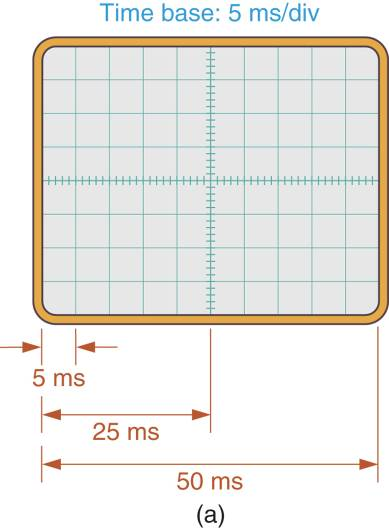Figure 8: Oscilloscope Time Measurement

Figure 8b shows how the period of a sine wave is measured with an oscilloscope. As shown in the figure, one cycle of the AC waveform is 8 major divisions in length. If the time base control is set to 100 ms/dv, the sine wave period will be:

$T=\left( 8\text{ }divisions \right)\times \frac{100ms}{div}$

The frequency of a waveform can be determined by measuring its period and then calculating its frequency, as demonstrated in Example 4.

Example 4

What is the frequency of the sine wave in figure 8c?

Solution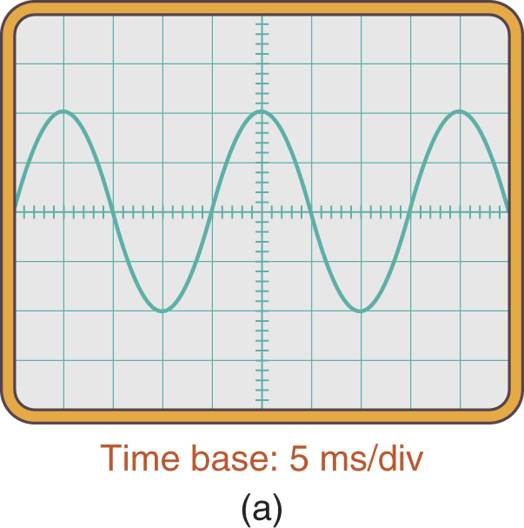Figure 8c

The display shows 2-1/2 cycles of a sine wave. Each cycle is 4 divisions in length. With a time base of 5 ms/div, the sine wave period is:

$T=\left( 4\text{ }divisions \right)\times \frac{5ms}{div}=20ms$

Once the sine wave period is known, the waveform frequency is found as:

$f=\frac{1}{T}=\frac{1}{20ms}=50Hz$

Summary of Time Related Characteristics

You have now been introduced to the most common time-related AC characteristics. These characteristics (and terms) are summarized in Figure 9.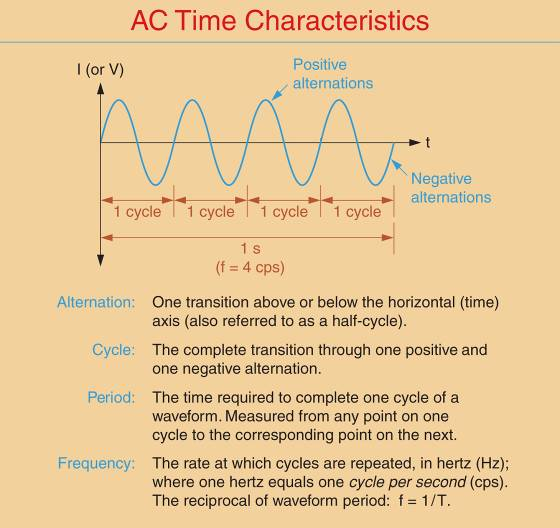Figure 9: AC time-related characteristics.

It should be noted that many electrical professionals do not use oscilloscopes on the job. Even so, the oscilloscope display provides a convenient means of illustrating many waveforms characteristics.

Summary

1. Alternating current is any current that changes direction periodically.
2. A waveform is a graph of the relationship between amplitude and time.
3. There are two alternations per cycle, one positive and one negative.
4. The period of a waveform is the time required to complete one complete cycle. It is also known as cycle time.
5. The period can be measured from any point on a waveform to the identical point on the next cycle.
6. Frequency is the rate at which waveform cycles repeat, in cycles per second (cps). The unit of measure is the hertz (Hz).
7. The period is equal to the reciprocal of frequency and vice versa. T = 1/f and f = 1/T.
8. An oscilloscope is a piece of test equipment that provides a visual display of a waveform.
9. The value of each major horizontal division on the oscilloscope display changes when you adjust the time base control.
10. Waveform period is measured by multiplying the time base by the number of horizontal divisions that a complete cycle of a waveform occupies on the oscilloscope display. The frequency can now be determined by calculating the reciprocal of the period.
Did you find apk for android? You can find new Free Android Games and apps.